# Best statistics for data science book to the people of all levels who want to reach the depth of statistics and data science.

Statistical methods play a key role in data science. There are some excellent introductory and advanced level textbooks for data scientists which explains how to apply various statistical methods to data science, how to avoid their misuse and gives you advice on what's important and what's not.

Here you will get some of the best statistics for data science books.

## 1. Naked Statistics: Stripping the Dread from the Data

This is a great informative book for those who are newer, and a little more experienced. This is a good introduction to practical statistics which provided a number of excellent practical logical explanations.  People who are interested in statistics and data science find this book very helpful.

Charles Wheelan clarifies key concepts such as

• Inference
• Correlation
• Regression analysis
• Randomized experiments
• Hypothesis tests
• Issues related to confidence level and p-value.

The writer reveals how biased or careless parties can manipulate or misrepresent data. He again shows us how brilliant and creative researchers are exploiting the valuable data from natural experiments to tackle thorny questions.

## 2. Statistics (the Easier Way) with R, 3rd Ed: an informal text on statistics and data science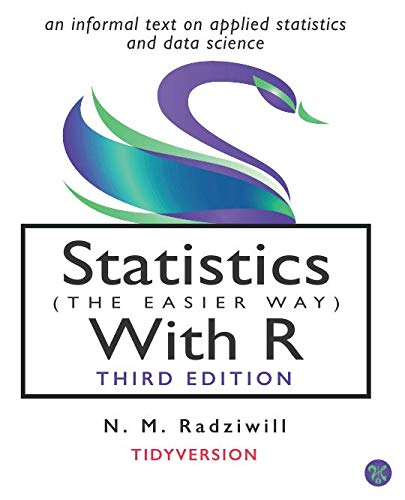Author: N M Radziwill,M C Benton
Published at: 20/04/2019
ISBN: 0996916032

Statistics with R is a great book for beginning data analysis. A beginner will quickly be able to use data analysis tools such as ggplot2 and Dplyr etc. Students and working professional find this book very informative. It provides an integrated treatment of statistical inference techniques in data science using the R statistical software.

So we can say that this is an awesome resource for all levels who want to reach the depth of statistics and data science.

## 3. An Introduction to Statistical Learning: with Applications in R (Springer Texts in Statistics)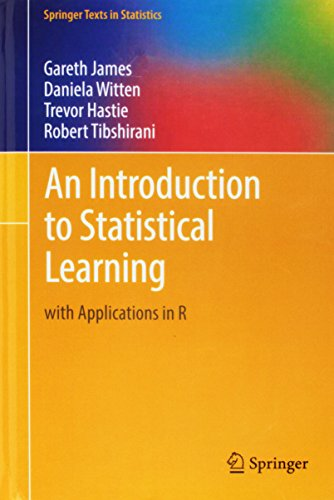Author: Gareth James,Daniela Witten,Trevor Hastie,Robert Tibshirani
Published at: 01/09/2017
ISBN: 1461471370

An Introduction to Statistical Learning provides you the right amount of theory and practice. This statistics for data science book requires no prior knowledge of calculus or linear algebra though it is an outstanding introduction to statistical learning.

This book provides you

• An accessible overview of the field of statistical learning
• An essential toolset for making sense of the vast and complex data sets that have emerged in fields ranging from biology to finance to marketing to astrophysics
• Some of the most important modeling and prediction techniques, along with relevant applications
• Linear regression
• Classification
• Resampling methods
• Shrinkage approaches
• Tree-based methods
• Support vector machines, clustering, and more

Each chapter contains a tutorial on implementing the analyses and methods presented in R, an extremely popular open-source statistical software platform.

## 4. Practical Statistics for Data Scientists: 50 Essential Concepts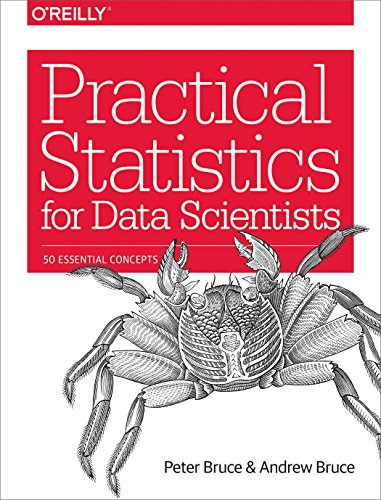Author: Peter Bruce,Andrew Bruce
Published at: 28/05/2017
ISBN: 1491952962

Practical Statistics for Data Scientists is an excellent introductory textbook for data scientists which explains how to apply various statistical methods to data science, how to avoid their misuse, and gives you advice on what's important and what's not. This is a good reference book as all the explanations are very clear.

This book includes

• Python code
• The curse of dimensionality
• A discussion of neural networks.

You’ll learn from this book

• Why exploratory data analysis is a key preliminary step in data science
• How random sampling can reduce bias and yield a higher quality dataset, even with big data
• How the principles of experimental design yield definitive answers to questions
• How to use regression to estimate outcomes and detect anomalies
• Key classification techniques for predicting which categories a record belongs to
• Statistical machine learning methods that learn from data
• Unsupervised learning methods for extracting meaning from unlabeled data

## 5. Statistical Rethinking: A Bayesian Course with Examples in R and Stan (Chapman & Hall/CRC Texts in Statistical Science)

Statistical Rethinking: A Bayesian Course with Examples in R and Stan is a nice and short introduction to statistical modeling. In this book, the author includes the basics of regression to multilevel models. He also discusses measurement error, missing data, and Gaussian process models for spatial and network autocorrelation.

Coverage includes

• The Golem of Prague
• Small worlds and large worlds
• Sampling the imaginary
• Linear models
• Multivariate linear models
• Overfitting and model comparison
• Interactions
• Markov chain Monte Carlo Estimation
• Big entropy and the generalized linear model
• Counting and classification
• Monsters and mixtures
• Multilevel models
• Adventures in covariance
• Missing data and other opportunities
• Horoscopes.

## 6. Statistics: The Art and Science of Learning from Data (4th Edition)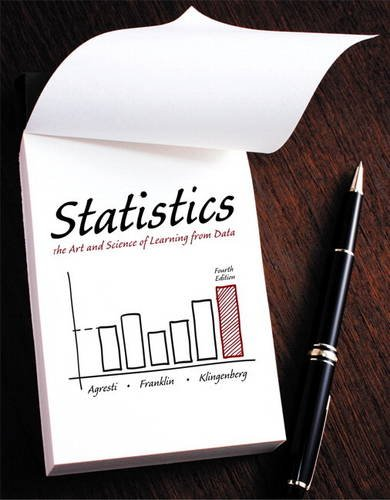Author: Alan Agresti,Christine A. Franklin,Bernhard Klingenberg
Published at: 13/01/2016
ISBN: 0321997832
Statistics: The Art and Science of Learning from Data includes a chapter summary and chapter problems at the end of every chapter. It includes an online review for a better understanding of the topics. It also explores data with graphs and numerical summaries, the association between two categorical variables, two quantitative variables, good and poor ways to sample and experiment, probability distributions and much much more.

This book is divided into four parts. These are

• Gathering and exploring data
• Probability, probability distribution, and  sampling distributions
• Inferential statistics
• Analyzing association and extended statistical method

It’s important for students to learn and analyze both quantitative and categorical data. Concepts are introduced first with categorical data, and then with quantitative data.

## 7. R Cookbook: Proven Recipes for Data Analysis, Statistics, and Graphics (O'reilly Cookbooks)

R Cookbook comes with more than 200 practical recipes. It provides a comprehensive reference on R where writing and explanations are good. This book is for the beginner as well as for intermediate users. After reading this book, you'll be able to create vectors, handle variables, and perform other basic functions. You can tackle data structures such as matrices, lists, factors, and data frames. Again create a variety of graphic displays.

The key terms are

• Navigating the software
• Input and output
• Data structures
• Data transformations
• Strings and date
• Probability
• General statistics
• Graphics
• Linear regression an ANOVA
• Useful tricks
• Beyond basic numerics and statistics
•   Time series analysis.

## 8. An Introduction to Statistics and Data Analysis Using Stata®: From Research Design to Final Report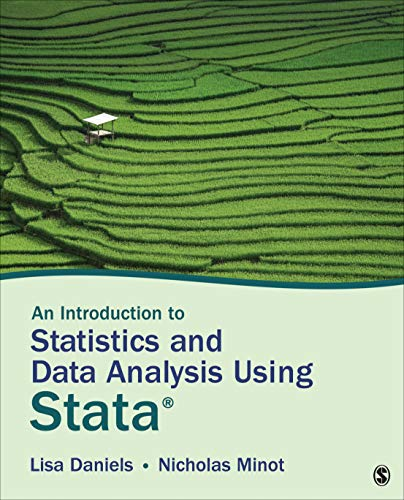Author: Lisa Daniels,Nicholas W. Minot
Published at: 29/01/2019
ISBN: 1506371833

This is a great book with excellent data about data analysis. It provides useful advice that applies in the real world jobs and techniques. This book is divided into five parts which are the research process and data collection, describing data, testing hypotheses, exploring relationships, and writing a research paper.

Key coverage is

• The research process
• Sampling techniques
• Questionnaire design
• An introduction to Stata
• Preparing and transforming your data
• Descriptive statistics
• The normal distributions
• Linear regression analysis and diagnostics
• Regression analysis with categorical dependent variables.

Thanks for reading this post. If you have any opinion don't hesitate to comment here. Also please subscribe our newsletter to get more updates.